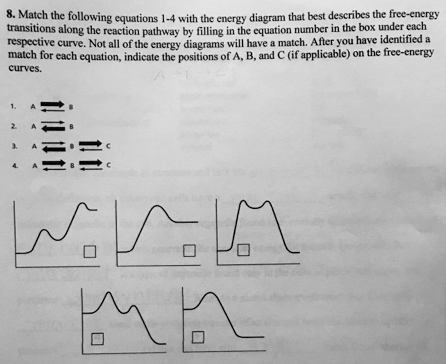# Problem: Match the following equations 1-4 with the energy diagram that best describes the free-energy transitions along the reaction pathway by filling in the equation number in the box under each respective curve. After you have identified a match for each equation, indicate the positions of A, B, and C (if applicable) on the free-energy curves.

###### FREE Expert Solution

1. This reaction contains a single energy transition (A to B), and is expected to have a single peak for its energy diagram. And since the reversible reaction shows preference to the formation of B (as evidenced by a broader forward arrow),  it is highly likely that substance B is more stable, and thus, would have a lower energy compared to A (i.e. energy level on left is higher than on the right).

2. This is another single energy transition reaction. However, unlike reaction 1, this reaction prefers the formation of A from B. Thus, substance A is most likely more stable, and would have a lower energy (i.e. energy level on the left is lower than on the right).###### Problem Details

Match the following equations 1-4 with the energy diagram that best describes the free-energy transitions along the reaction pathway by filling in the equation number in the box under each respective curve.

After you have identified a match for each equation, indicate the positions of A, B, and C (if applicable) on the free-energy curves.